## 《高等数学(1)》21春平时作业3

《高等数学(1)》21春平时作业3

------------------------
1.设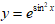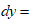（ ）
A.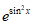B.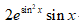C.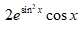D.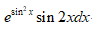2.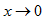时，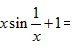（ ）
A.无穷大量
B.无穷小量
C.有界变量
D.无界变量

3.题面见图片A.A
B.B
C.C
D.D

4.题面见图片A.A
B.B
C.C
D.D

5.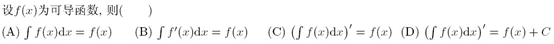A.(A)
B.(B)
C.(C)
D.(D)

6.题面见图片A.A
B.B
C.C
D.D

7.A.(A)
B.(B)
C.(C)
D.(D)

8.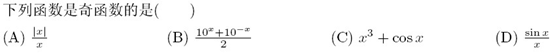A.(A)
B.(B)
C.(C)
D.(D)

9.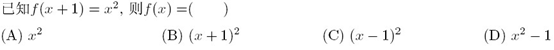A.(A)
B.(B)
C.(C)
D.(D)

10.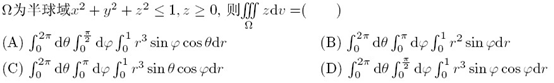A.(A)
B.(B)
C.(C)
D.(D)

11.已知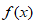是偶函数，当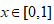时，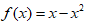，则当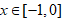时，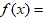（ ）
A.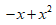B.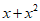C.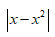D.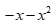12.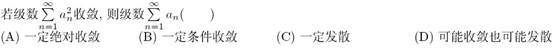A.(A)
B.(B)
C.(C)
D.(D)

13.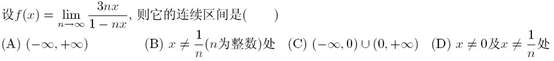A.(A)
B.(B)
C.(C)
D.(D)

14.题面见图片A.A
B.B
C.C
D.D

15.已知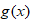均为定义域D上的奇函数则下列说法中正确的是（ ）
A.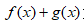为奇函数
B.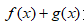为偶函数
C.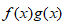为奇函数
D.为偶函数

16.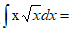（ ）
A.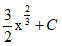B.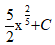C.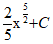D.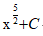17.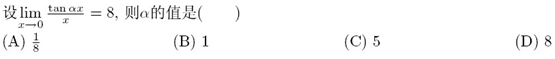A.(A)
B.(B)
C.(C)
D.(D)

18.若函数f(x)满足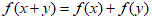，则f(x)是（ ）
A.偶函数
B.非奇非偶函数
C.奇函数
D.奇偶性不确定

19.题面见图片A.A
B.B
C.C
D.D

20.题面见图片A.A
B.B
C.C
D.D